# Replication Codes for Experimental Evaluation of Algorithm-Assisted Human Decision-Making: Application to Pretrial Public Safety Assessment

library(aihuman)

Imai, Kosuke, Zhichao Jiang, D. James Greiner, Ryan Halen, and Sooahn Shin. “Experimental Evaluation of Algorithm-Assisted Human Decision-Making: Application to Pretrial Public Safety Assessment.” (with discussion) Journal of the Royal Statistical Society, Series A (Statistics in Society), 2023.

The data used for this paper, and made available here, are interim, based on only half of the observations in the study and (for those observations) only half of the study follow-up period. We use them only to illustrate methods, not to draw substantive conclusions.

## Overview

The main functions in this package are as follows:

Category Function Type Main Input Output Paper Notes
descriptive PlotStackedBar() vis. e.g., data(psa_synth) ggplot Fig 1 dist. of $$D_i$$
descriptive CalDIMsubgroup() est. e.g., data(synth) dataframe Sec 2.4 diff-in-means
descriptive PlotDIMdecisions() vis. output of CalDIMsubgroup() ggplot Fig 2 (left)
descriptive PlotDIMoutcomes() vis. output of CalDIMsubgroup() for each outcome ggplot Fig 2 (right)
main AiEvalmcmc() est.(c/h) e.g., data(synth) mcmc Sec S5
main CalAPCE() est.(c/h) output of AiEvalmcmc() list Sec 3.4
main APCEsummary() est. output of CalAPCE() dataframe Sec 3.4 APCE
main PlotAPCE() vis. output of APCEsummary() ggplot Fig 4
strata CalPS() est. $P.R.mcmc of CalAPCE() dataframe Eq 6 $$e_r$$ strata PlotPS() vis. output of CalPS() ggplot Fig 3 fairness CalFairness() est. output of CalAPCE() dataframe Sec 3.6 $$\Delta_r(z)$$ fairness PlotFairness() est. output of CalFairness() ggplot Fig 5 optimal CalOptimalDecision() est. output of AiEvalmcmc() dataframe Sec 3.7 $$\delta^\ast(\mathbf{x})$$ optimal PlotOptimalDecision() vis. output of CalOptimalDecision() ggplot Fig 6 comparison PlotUtilityDiff() vis. output of CalOptimalDecision() ggplot Fig 7 $$g_d(\mathbf{x})$$ comparison PlotUtilityDiffCI() vis. output of CalOptimalDecision() ggplot Fig S17 crt SpilloverCRT() est. court event hearing date, $$D_i$$, $$Z_i$$ daraframe Sec S3.1 crt PlotSpilloverCRT() vis. output of SpilloverCRT() ggplot Fig S8 crt power SpilloverCRTpower() est. court event hearing date, $$D_i$$, $$Z_i$$ dataframe Sec S3.2 crt power PlotSpilloverCRTpower() vis. output of SpilloverCRTpower() ggplot Fig S9 frequentist CalAPCEipw() est. e.g., data(synth) dataframe Sec S7 frequentist BootstrapAPCEipw() est. e.g., data(synth) dataframe frequentist APCEsummaryipw() est. outputs of CalAPCEipw() and BootstrapAPCEipw() dataframe frequentist (RE) CalAPCEipwRE() est. e.g., data(synth) dataframe frequentist (RE) BootstrapAPCEipwRE() est. e.g., data(synth) dataframe vis. = visualization; est. = estimation; c/h = computation-heavy. You may use CalAPCEparallel() instead of CalAPCE() throughout the analysis. ## Synthetic data In this document, we will use synthetic data for the analysis. We have three different versions of data, depending on whether it includes PSA variables (FTA score, NCA score, NVCA flag, and DMF recommendation) or court event hearing date. ### Data 1: synth This is the main data that will be used for estimating Average Principal Causal Effects (APCE). The data should be in form of data.frame, and must contain the following columns: • Z: A binary treatment (PSA provision) • D: An ordinal decision (judge’s release decision) • Y: An outcome (FTA or NCA or NVCA) • Pre-treatment covariates Note that the column names of treatment, decision, and outcome should be specified as “Z”, “D”, and “Y” respectively. In this example, we assume four ordinal decisions (i.e., $$D_i = 0, 1, 2, 3$$). See Section 3.4 of the paper for more details. data("synth") str(synth) #> 'data.frame': 1000 obs. of 11 variables: #>$ Y                           : int  0 0 0 0 0 0 1 0 1 0 ...
#>  $D : int 0 0 1 0 2 0 0 0 0 0 ... #>$ Z                           : int  0 1 0 0 0 0 0 0 0 1 ...
#>  $Sex : int 0 1 1 1 1 1 1 1 1 1 ... #>$ White                       : int  0 1 1 0 1 0 0 0 1 1 ...
#>  $Age : int 28 19 33 38 27 25 22 34 39 34 ... #>$ CurrentViolentOffense       : int  0 1 0 0 0 0 1 1 1 0 ...
#>  $PendingChargeAtTimeOfOffense: int 0 0 0 0 1 0 1 0 1 1 ... #>$ PriorMisdemeanorConviction  : int  1 1 1 0 1 1 0 1 1 0 ...
#>  $PriorFelonyConviction : int 0 0 1 0 1 1 0 0 1 0 ... #>$ PriorViolentConviction      : int  3 0 0 0 0 1 0 2 0 0 ...
synth[1:6,]
#>   Y D Z Sex White Age CurrentViolentOffense PendingChargeAtTimeOfOffense
#> 1 0 0 0   0     0  28                     0                            0
#> 2 0 0 1   1     1  19                     1                            0
#> 3 0 1 0   1     1  33                     0                            0
#> 4 0 0 0   1     0  38                     0                            0
#> 5 0 2 0   1     1  27                     0                            1
#> 6 0 0 0   1     0  25                     0                            0
#>   PriorMisdemeanorConviction PriorFelonyConviction PriorViolentConviction
#> 1                          1                     0                      3
#> 2                          1                     0                      0
#> 3                          1                     1                      0
#> 4                          0                     0                      0
#> 5                          1                     1                      0
#> 6                          1                     1                      1
unique(synth$D) #>  0 1 2 3 ### Data 2: psa_synth This is a data.frame that consists of synthetic Z, D, and four PSA variables (FTAScore, NCAScore, NVCAFlag, and DMF). This will be used for PlotStackedBar() function (e.g., Figure 1). data("psa_synth") str(psa_synth) #> tibble [1,000 × 6] (S3: tbl_df/tbl/data.frame) #>$ Z       : int [1:1000] 0 1 0 0 0 0 0 0 0 1 ...
#>  $D : int [1:1000] 0 0 1 0 2 0 0 0 0 0 ... #>$ FTAScore: num [1:1000] 1 4 2 3 1 3 3 3 2 2 ...
#>  $NCAScore: num [1:1000] 1 5 3 3 1 3 3 3 3 3 ... #>$ NVCAFlag: num [1:1000] 0 0 0 0 0 0 0 0 0 0 ...
#>  $DMF : num [1:1000] 0 1 1 0 0 0 0 0 0 0 ... psa_synth[1:6,] #> # A tibble: 6 × 6 #> Z D FTAScore NCAScore NVCAFlag DMF #> <int> <int> <dbl> <dbl> <dbl> <dbl> #> 1 0 0 1 1 0 0 #> 2 1 0 4 5 0 1 #> 3 0 1 2 3 0 1 #> 4 0 0 3 3 0 0 #> 5 0 2 1 1 0 0 #> 6 0 0 3 3 0 0 ### Data 3: hearingdate_synth This is a Date vector that includes synthetic court event hearing date for each cases. It will be used for testing the potential existence of spillover effects: SpilloverCRT(). See Appendix S3 for more details. data("hearingdate_synth") str(hearingdate_synth) #> Date[1:1000], format: "2000-06-01" "2000-06-02" "2000-06-02" "2000-06-02" "2000-06-02" ... hearingdate_synth[1:6] #>  "2000-06-01" "2000-06-02" "2000-06-02" "2000-06-02" "2000-06-02" #>  "2000-06-02" ### Interim data In the original paper, we use three data.frame of which Y corresponds to each of three binary outcome variables – failure to appear (FTA), new criminal activity (NCA), and new violent criminal activity (NVCA).For example, FTAdata consists of following columns: • Z: A binary treatment (PSA provision) • D: An ordinal decision (judge’s release decision) • Y: An outcome (FTA) • Pre-treatment covariates: Sex, White, SexWhite, Age, NCorNonViolentMisdemeanorCharge, ViolentMisdemeanorCharge, NonViolentFelonyCharge, ViolentFelonyCharge, PendingChargeAtTimeOfOffense, PriorMisdemeanorConviction, PriorFelonyConviction, PriorViolentConviction, PriorSentenceToIncarceration, PriorFTAInPastTwoYears, PriorFTAOlderThanTwoYears, and Staff_ReleaseRecommendation. See the table below for the details of the pre-treatment covariates: Variables Description Sex male or female White white or non-white SexWhite the interaction between Sex and White Age age NCorNonViolentMisdemeanorCharge binary variable for current NC (parole violations) or non-violent misdemeanor charge ViolentMisdemeanorCharge binary variable for current violent misdemeanor charge NonViolentFelonyCharge binary variable for current non-violent felony charge ViolentFelonyCharge binary variable for current violent felony charge PendingChargeAtTimeOfOffense binary variable for pending charge (felony, misdemeanor, or both) at the time of offense PriorMisdemeanorConviction binary variable for prior conviction of misdemeanor PriorFelonyConviction binary variable for prior conviction of felony PriorViolentConviction four-level ordinal variable for prior violent conviction ($$0,1,2$$ and $$3$$, where $$3$$ indicates the counts of three or more) PriorSentenceToIncarceration binary variable for prior sentence to incarceration PriorFTAInPastTwoYears three-level ordinal variable for FTAs from past two years ($$0,1$$ and $$2$$ where $$2$$ indicates the counts of two or more) PriorFTAOlderThanTwoYears binary variable for FTAs from over two years ago Staff_ReleaseRecommendation four-level ordinal variable for the DMF recommendation Additionally, PSAdata is a data.frame that consists of Z, D, and four PSA variables (FTAScore, NCAScore, NVCAFlag, and DMF). This will be used for PlotStackedBar() function (e.g., Figure 1). Lastly, HearingDate is a factor vector for court event hearing date for each cases. It will be used for testing the potential existence of spillover effects: SpilloverCRT(). See Appendix S3 for more details. The data used for this paper, and made available here, are interim, based on only half of the observations in the study and (for those observations) only half of the study follow-up period. We use them only to illustrate methods, not to draw substantive conclusions. ## Descriptive statistics Before we get into the main analysis, we can visualize some descriptive statistics using the following functions: • PlotStackedBar(): The distribution of the judge’s decisions given the pretrial public safety assessment (PSA). See Figure 1 for example. • CalDIMsubgroup(), PlotDIMdecisions(), and PlotDIMoutcomes() ### Distribution of the judge’s decisions (Figure 1) PlotStackedBar() plots the distribution of the judge’s decisions given the PSA. Similarly, PlotStackedBarDMF() plots the distribution of the judge’s decisions given the DMF recommendation. By using the subset of the data, you may also plot the distribution among particular cases (e.g., treatment group, female arrestees). See Figure 1 and Appendix S1 for example. Note that the function requires a data.frame of which columns includes an ordinal decision (D), and psa variables (fta, nca, and nvca) as in data(psa_synth). # Call the data data(psa_synth) # Treated group (PSA provided) p.treated = PlotStackedBar(psa_synth[psa_synth$Z == 1, ],
d.colors = c("grey80", "grey60", "grey30", "grey10"),
d.labels = c("signature","small","middle","large"),
legend.position = "bottom")
# Control group (PSA not provided)
p.control = PlotStackedBar(psa_synth[psa_synth$Z == 0, ], d.colors = c("grey80", "grey60", "grey30", "grey10"), d.labels = c("signature","small","middle","large"), legend.position = "bottom") p.dmf = PlotStackedBarDMF(psa_synth, d.colors = c("grey80", "grey60", "grey30", "grey10"), d.labels = c("signature","small","middle","large"), dmf.label = "DMF") # For more details # ?PlotStackedBar # Example p.treated$p.fta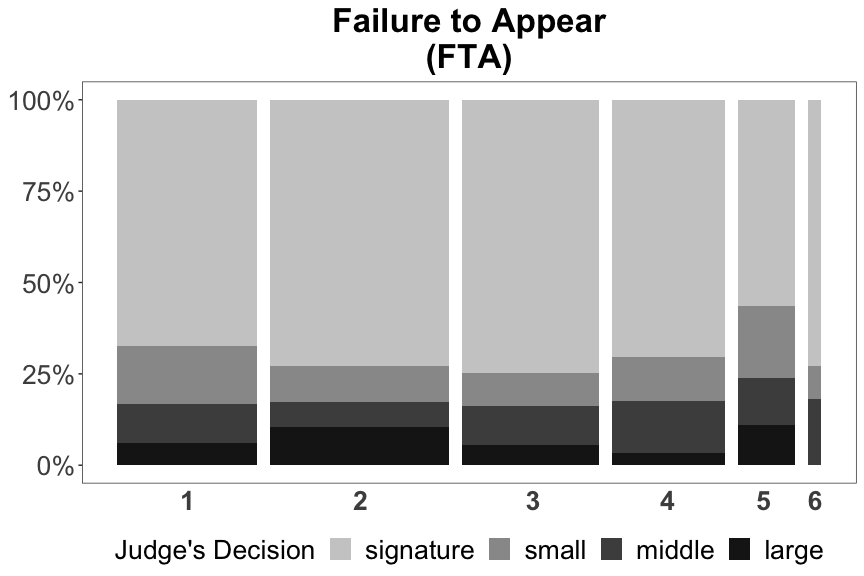You may plot the three figures (each for FTA, NCA, and NVCA) together using the codes below.

# To plot three figures together
library(ggplot2)
library(gridExtra)

mylegend <- g_legend(p.control$p.nvca) # Treated grid.arrange(arrangeGrob(p.control$p.fta + theme(legend.position="none"),
p.control$p.nca + theme(legend.position="none"), p.control$p.nvca + theme(legend.position="none"),
p.dmf$p.treat + theme(legend.position="none"), nrow=1), mylegend, nrow=2,heights=c(10, 1)) # Control grid.arrange(arrangeGrob(p.control$p.fta + theme(legend.position="none"),
p.control$p.nca + theme(legend.position="none"), p.control$p.nvca + theme(legend.position="none"),
p.dmf$p.control + theme(legend.position="none"), nrow=1), mylegend, nrow=2,heights=c(10, 1)) #### Replication codes See the codes that are used to plot Figure 1 and Figure S1-S3 below. library(aihuman) data(PSAdata) library(tidyverse) library(gridExtra) # Figure 1 p.treated = PlotStackedBar(PSAdata %>% filter(Z==1), legend.position = "bottom") p.control = PlotStackedBar(PSAdata %>% filter(Z==0), legend.position = "bottom") p.dmf = PlotStackedBarDMF(PSAdata, dmf.category = c("signature bond", "cash bond")) mylegend = g_legend(p.treated$p.nvca)
pdf("figs/stackedbar.pdf", height = 6, width = 22)
grid.arrange(arrangeGrob(p.treated$p.fta + theme(legend.position="none"), p.treated$p.nca + theme(legend.position="none"),
p.treated$p.nvca + theme(legend.position="none"), p.dmf$p.treat + theme(legend.position="none"),
nrow=1),
mylegend, nrow=2,heights=c(10, 1))
dev.off()
pdf("figs/stackedbar_control.pdf", height = 6, width = 22)
grid.arrange(arrangeGrob(p.control$p.fta + theme(legend.position="none"), p.control$p.nca + theme(legend.position="none"),
p.control$p.nvca + theme(legend.position="none"), p.dmf$p.control + theme(legend.position="none"),
nrow=1),
mylegend, nrow=2,heights=c(10, 1))
dev.off()

# Figure S1
S0.t = PlotStackedBar(PSAdata %>% filter(Z==1&Sex==0), legend.position = "bottom")
S0.c = PlotStackedBar(PSAdata %>% filter(Z==0&Sex==0), legend.position = "bottom")
dmf.category = c("signature bond", "cash bond"))
pdf("figs/stackedbar_F.pdf", height = 6, width = 22)
grid.arrange(arrangeGrob(S0.t$p.fta + theme(legend.position="none"), S0.t$p.nca + theme(legend.position="none"),
S0.t$p.nvca + theme(legend.position="none"), S0.dmf$p.treat + theme(legend.position="none"),
nrow=1),
mylegend, nrow=2,heights=c(10, 1))
dev.off()
pdf("figs/stackedbar_control_F.pdf", height = 6, width = 22)
grid.arrange(arrangeGrob(S0.c$p.fta + theme(legend.position="none"), S0.c$p.nca + theme(legend.position="none"),
S0.c$p.nvca + theme(legend.position="none"), S0.dmf$p.control + theme(legend.position="none"),
nrow=1),
mylegend, nrow=2,heights=c(10, 1))
dev.off()

# Figure S3
S1W1.t = PlotStackedBar(PSAdata %>% filter(Z==1&Sex==1&White==1), legend.position = "bottom")
S1W1.c = PlotStackedBar(PSAdata %>% filter(Z==0&Sex==1&White==1), legend.position = "bottom")
dmf.category = c("signature bond", "cash bond"))
pdf("figs/stackedbar_WM.pdf", height = 6, width = 22)
grid.arrange(arrangeGrob(S1W1.t$p.fta + theme(legend.position="none"), S1W1.t$p.nca + theme(legend.position="none"),
S1W1.t$p.nvca + theme(legend.position="none"), S1W1.dmf$p.treat + theme(legend.position="none"),
nrow=1),
mylegend, nrow=2,heights=c(10, 1))
dev.off()
pdf("figs/stackedbar_control_WM.pdf", height = 6, width = 22)
grid.arrange(arrangeGrob(S1W1.c$p.fta + theme(legend.position="none"), S1W1.c$p.nca + theme(legend.position="none"),
S1W1.c$p.nvca + theme(legend.position="none"), S1W1.dmf$p.control + theme(legend.position="none"),
nrow=1),
mylegend, nrow=2,heights=c(10, 1))
dev.off()

### Intention to treat effects of PSA Provision (Figure 2)

PlotDIMdecisions() plots estimated average causal effects of PSA provision on the judge’s decisions and PlotDIMoutcomes() plots that on the outcome. The results are based on the difference-in-means estimator. Please check Figure 2 for example. To plot these, you may need to compute the estimates using CalDIMsubgroup() function first. This function requires the following main arguments:

• data: A data.frame of which columns includes a binary treatment (Z), an ordinal decision (D), and an outcome variable (Y) as in data(synth).
• subgroup: A list of numeric vectors for the index of each of the five subgroups.

See the example below:

# Call the data
data(synth)

# Subgroup index
subgroup_synth = list(1:nrow(synth),
which(synth$Sex==0), which(synth$Sex==1),
which(synth$Sex==1&synth$White==0),
which(synth$Sex==1&synth$White==1))

# Compute Diff-in-Means on decision
res_dec = CalDIMsubgroup(synth, subgroup = subgroup_synth)
# Plot the results
PlotDIMdecisions(res_dec,
decision.labels = c("signature","small cash","middle cash","large cash"),
col.values = c("grey60", "grey30", "grey6", "grey1"),
shape.values = c(16, 17, 15, 18))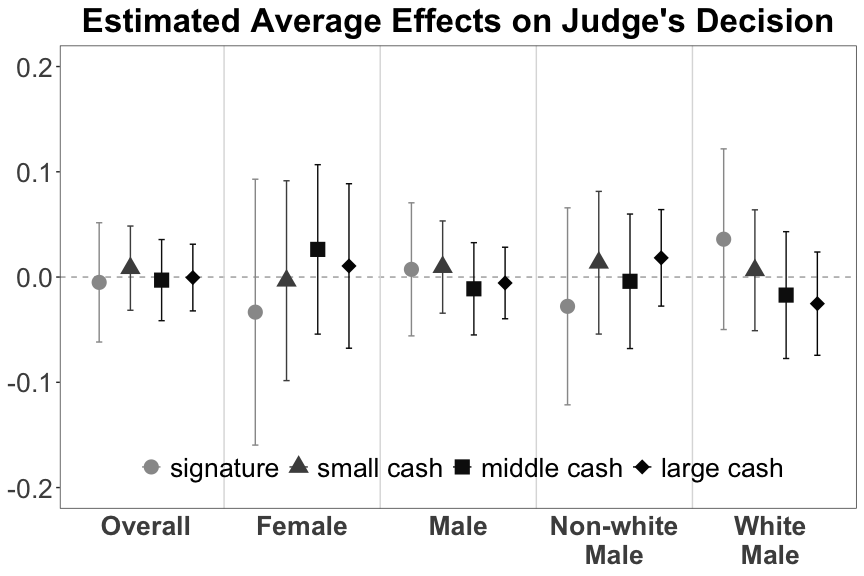# Synthetic data for outcome
synth_fta <- synth_nca <- synth_nvca <- synth
set.seed(123)
synth_fta$Y <- sample(0:1, 1000, replace = T) synth_nca$Y <- sample(0:1, 1000, replace = T)
synth_nvca$Y <- sample(0:1, 1000, replace = T) # Compute Diff-in-Means on outcome res_fta = CalDIMsubgroup(synth_fta, subgroup = subgroup_synth) res_nca = CalDIMsubgroup(synth_nca, subgroup = subgroup_synth) res_nvca = CalDIMsubgroup(synth_nvca, subgroup = subgroup_synth) # Plot the results PlotDIMoutcomes(res_fta, res_nca, res_nvca, y.max = 0.3)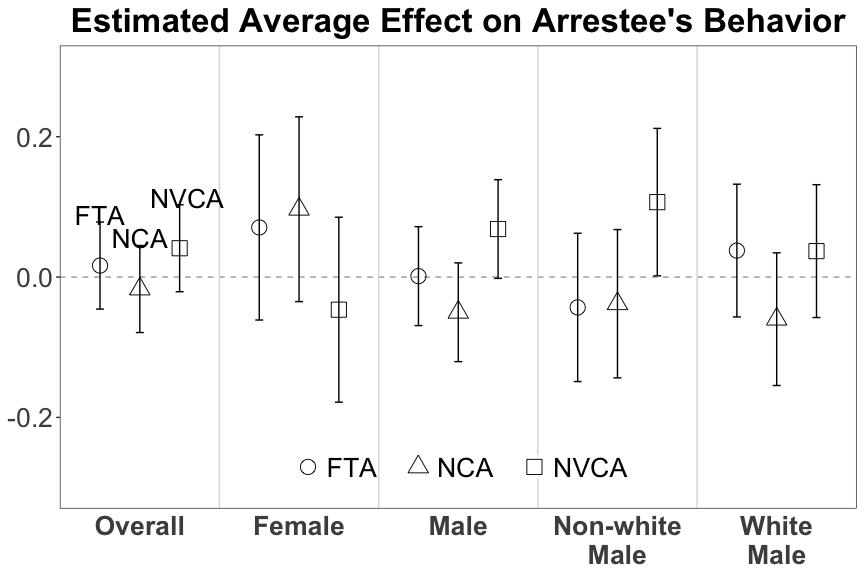#### Replication codes See the codes that are used to plot Figure 2 below. # Figure 2 data(FTAdata) data(NCAdata) data(NVCAdata) # Subgroup index subgroup_data = list(1:nrow(FTAdata), which(FTAdata$Sex==0),
which(FTAdata$Sex==1), which(FTAdata$Sex==1&FTAdata$White==0), which(FTAdata$Sex==1&FTAdata$White==1)) # Compute Diff-in-Means on decision res_dec = CalDIMsubgroup(FTAdata, subgroup = subgroup_data) # Plot the results pdf("figs/itt_decisions.pdf", width = 9, height = 5) PlotDIMdecisions(res_dec, y.max = 0.17) dev.off() # Compute Diff-in-Means on outcome res_fta = CalDIMsubgroup(FTAdata, subgroup = subgroup_data) res_nca = CalDIMsubgroup(NCAdata, subgroup = subgroup_data) res_nvca = CalDIMsubgroup(NVCAdata, subgroup = subgroup_data) # Plot the results pdf("figs/itt_outcomes.pdf", width = 9, height = 5) PlotDIMoutcomes(res_fta, res_nca, res_nvca, y.max = 0.17, top.margin = 0.02, bottom.margin = 0.02, label.position = c("top", "bottom", "top")) dev.off() For subgroup analysis for age groups in Figure S5: # Figure S5 # Subgroup index subgroup_age = list(which(FTAdata$Age<23),
which(FTAdata$Age>=23&FTAdata$Age<29),
which(FTAdata$Age>=29&FTAdata$Age<36),
which(FTAdata$Age>=36&FTAdata$Age<46),
which(synth$Sex==1), which(synth$Sex==1&synth$White==0), which(synth$Sex==1&synth$White==1)) sample_apce = CalAPCE(data = synth, mcmc.re = sample_mcmc, subgroup = subgroup_synth) # Or using parallel computing (results are the same): # sample_apce = CalAPCEparallel(data = synth, # mcmc.re = sample_mcmc, # subgroup = subgroup_synth, # burnin = 0, # size = 2) ### APCEsummary() APCEsummary() computes the average and 95% quantile of APCE of each sample. You only need the output of CalAPCE() or CalAPCEparallel(). sample_apce_summary = APCEsummary(sample_apce[["APCE.mcmc"]]) ### PlotAPCE() (Figure 4) PlotAPCE() visualizes the results as in Figure 4. See the example below: PlotAPCE(sample_apce_summary, y.max = 0.25, decision.labels = c("signature","small cash","middle cash","large cash"), shape.values = c(16, 17, 15, 18), col.values = c("blue", "black", "red", "brown", "purple"), label = FALSE)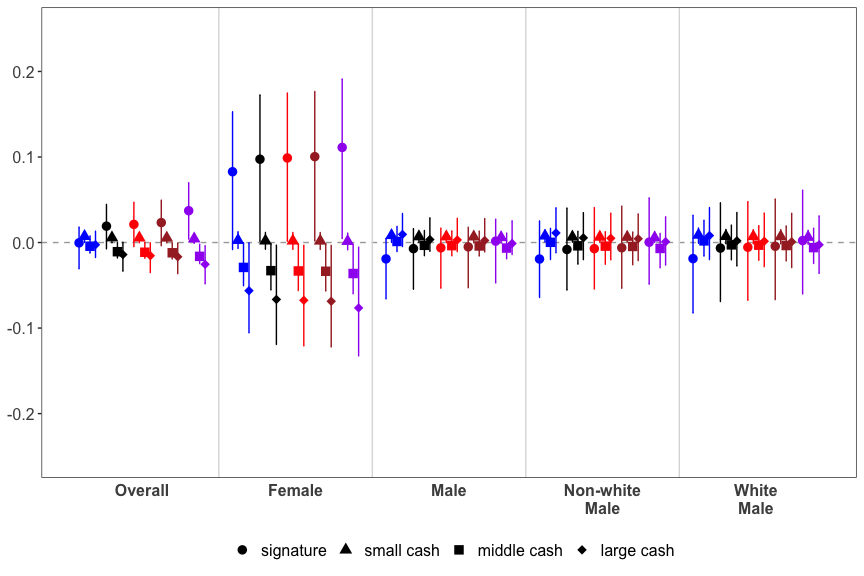### Replication codes The codes below is used for the main analysis and the visualization of Figure 4: ## Main analysis # MCMC library(coda) PSADiag_ordinal = function(data, rho=0, ## mcmc parameters n.mcmc = 5*10^4, verbose = TRUE, out.length = 500){ set.seed(111) mcmc1 = AiEvalmcmc(data,rho=rho,n.mcmc=n.mcmc,verbose=TRUE,out.length=out.length) set.seed(222) mcmc2 = AiEvalmcmc(data,rho=rho,n.mcmc=n.mcmc,verbose=TRUE,out.length=out.length) set.seed(333) mcmc3 = AiEvalmcmc(data,rho=rho,n.mcmc=n.mcmc,verbose=TRUE,out.length=out.length) set.seed(444) mcmc4 = AiEvalmcmc(data,rho=rho,n.mcmc=n.mcmc,verbose=TRUE,out.length=out.length) set.seed(555) mcmc5 = AiEvalmcmc(data,rho=rho,n.mcmc=n.mcmc,verbose=TRUE,out.length=out.length) mcmc.PSA = mcmc.list(mcmc1,mcmc2,mcmc3,mcmc4, mcmc5) return(mcmc.PSA) } PSA.ordinal.diag.fta = PSADiag_ordinal(FTAdata,n.mcmc=50000,verbose=TRUE,out.length=500) PSA.ordinal.diag.nca = PSADiag_ordinal(NCAdata,n.mcmc=50000,verbose=TRUE,out.length=500) PSA.ordinal.diag.nvca = PSADiag_ordinal(NVCAdata,n.mcmc=50000,verbose=TRUE,out.length=500) FTAmcmc <- lapply(PSA.ordinal.diag.fta, function(x) x[-(1: floor(0.5*dim(x))),]) FTAmcmc <- do.call("rbind", FTAmcmc) FTAmcmc <- mcmc(FTAmcmc) NCAmcmc <- lapply(PSA.ordinal.diag.nca, function(x) x[-(1: floor(0.5*dim(x))),]) NCAmcmc <- do.call("rbind", NCAmcmc) NCAmcmc <- mcmc(NCAmcmc) NVCAmcmc <- lapply(PSA.ordinal.diag.nvca, function(x) x[-(1: floor(0.5*dim(x))),]) NVCAmcmc <- do.call("rbind", NVCAmcmc) NVCAmcmc <- mcmc(NVCAmcmc) # APCE subg = list(1:nrow(FTAdata), which(FTAdata$Sex==0),
which(FTAdata$Sex==1), which(FTAdata$Sex==1&FTAdata$White==0), which(FTAdata$Sex==1&FTAdata$White==1)) FTAace <- CalAPCEparallel(FTAdata,FTAmcmc,subgroup=subg,rho=0,burnin=0,size=5) FTAqoi <- APCEsummary(FTAace[["APCE.mcmc"]]) NCAace <- CalAPCEparallel(NCAdata,NCAmcmc,subgroup=subg,rho=0,burnin=0,size=5) NCAqoi <- APCEsummary(NCAace[["APCE.mcmc"]]) NVCAace <- CalAPCEparallel(NVCAdata,NVCAmcmc,subgroup=subg,rho=0,burnin=0,size=5) NVCAqoi <- APCEsummary(NVCAace[["APCE.mcmc"]]) # Figure 4 pdf("figs/qoi_fta.pdf", width = 9, height = 3) PlotAPCE(FTAqoi, y.max = 0.166, top.margin = 0.05, bottom.margin = 0.05, label.position = c("top", "bottom", "top", "bottom")) + labs(title = "Failure to Appear (FTA)") + theme(legend.position = "none") dev.off() pdf("figs/qoi_nca.pdf", width = 9, height = 3) PlotAPCE(NCAqoi, y.max = 0.166, label = FALSE) + labs(title = "New Criminal Activity (NCA)") + theme(legend.position = "none") dev.off() pdf("figs/qoi_nvca.pdf", width = 9, height = 3.5) PlotAPCE(NVCAqoi, y.max = 0.166, label = FALSE) + labs(title = "New Violent Criminal Activity (NVCA)") dev.off() For subgroup analysis for age groups in Figure S7: # APCE subg.age = list(which(FTAdata$Age<23),
which(FTAdata$Age>=23&FTAdata$Age<29),
which(FTAdata$Age>=29&FTAdata$Age<36),
which(FTAdata$Age>=36&FTAdata$Age<46),
which(FTAdata$Age>=46)) subg.age.lab = c("17~22", "23~28", "29~35", "36~45", "46~") FTAace.age <- CalAPCEparallel(FTAdata,FTAmcmc,subgroup=subg.age, name.group=subg.age.lab, rho=0,burnin=0,size=5) FTAqoi.age <- APCEsummary(FTAace.age[["APCE.mcmc"]]) NCAace.age <- CalAPCEparallel(NCAdata,NCAmcmc,subgroup=subg.age, name.group=subg.age.lab, rho=0,burnin=0,size=5) NCAqoi.age <- APCEsummary(NCAace.age[["APCE.mcmc"]]) NVCAace.age <- CalAPCEparallel(NVCAdata,NVCAmcmc,subgroup=subg.age, name.group=subg.age.lab, rho=0,burnin=0,size=5) NVCAqoi.age <- APCEsummary(NVCAace.age[["APCE.mcmc"]]) # Figure S7 pdf("figs/qoi_fta_age.pdf", width = 9, height = 3) PlotAPCE(FTAqoi.age, y.max = 0.124, top.margin = 0.03, bottom.margin = 0.03, label.position = c("top", "bottom", "top", "bottom"), name.group = subg.age.lab) + labs(title = "Failure to Appear (FTA)") + theme(legend.position = "none") dev.off() pdf("figs/qoi_nca_age.pdf", width = 9, height = 3) PlotAPCE(NCAqoi.age, y.max = 0.124, label = FALSE, name.group = subg.age.lab) + labs(title = "New Criminal Activity (NCA)") + theme(legend.position = "none") dev.off() pdf("figs/qoi_nvca_age.pdf", width = 9, height = 3.5) PlotAPCE(NVCAqoi.age, y.max = 0.124, label = FALSE, name.group = subg.age.lab) + labs(title = "New Violent Criminal Activity (NVCA)") dev.off() ## Principal strata (Figure 3) Using one of the output ($P.R.mcmc) of CalAPCE() (or equivalently CalAPCEparallel()), CalPS() estimates the proportion of each principal stratum ($$R_i = 0, \ldots, k+1$$) and PlotPS() visualizes the results. See section 3.4 for more details about the principal strata in this context.

sample_ps = CalPS(sample_apce$P.R.mcmc) PlotPS(sample_ps, col.values = c("blue", "black", "red", "brown", "purple"), label = FALSE)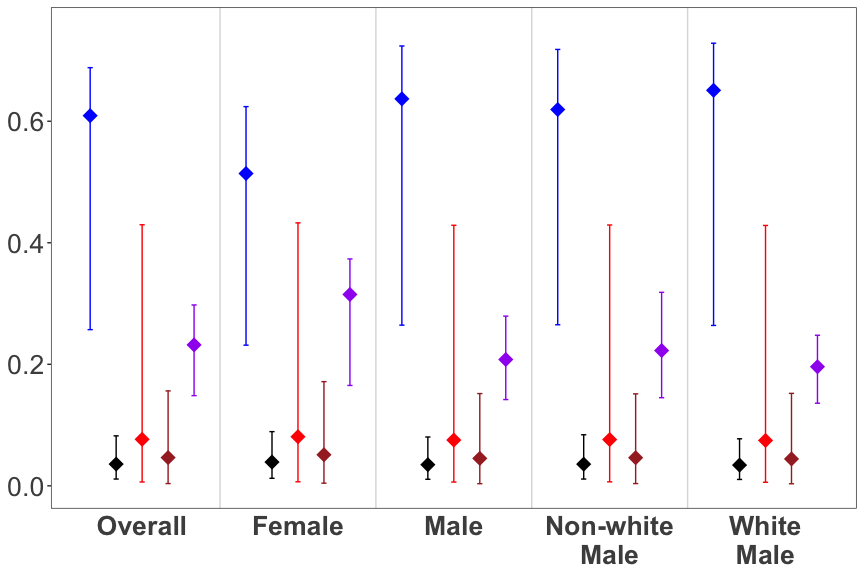### Replication codes To plot estimated proportion of each principal stratum as in Figure 3: # P.R ps_fta = CalPS(FTAace[["P.R.mcmc"]]) ps_nca = CalPS(NCAace[["P.R.mcmc"]]) ps_nvca = CalPS(NVCAace[["P.R.mcmc"]]) # Figure 3 pdf("figs/er_fta.pdf", width = 8, height = 6) PlotPS(ps_fta, y.max = 1, top.margin = 0.08, label.size = 5.2) + labs(title = "Failure to Appear (FTA)") dev.off() pdf("figs/er_nca.pdf", width = 8, height = 6) PlotPS(ps_nca, y.max = 1, top.margin = 0.08, label = FALSE) + labs(title = "New Criminal Activity (NCA)") dev.off() pdf("figs/er_nvca.pdf", width = 8, height = 6) PlotPS(ps_nvca, y.max = 1, top.margin = 0.08, label = FALSE) + labs(title = "New Violent Criminal Activity (NVCA)") dev.off() For subgroup analysis for age groups in Figure S7: # P.R ps_fta.age = CalPS(FTAace.age[["P.R.mcmc"]]) ps_nca.age = CalPS(NCAace.age[["P.R.mcmc"]]) ps_nvca.age = CalPS(NVCAace.age[["P.R.mcmc"]]) # Figure S7 pdf("figs/er_fta_age.pdf", width = 8, height = 6) PlotPS(ps_fta.age, y.max = 1, top.margin = 0.08, label.size = 5.2) + labs(title = "Failure to Appear (FTA)") dev.off() pdf("figs/er_nca_age.pdf", width = 8, height = 6) PlotPS(ps_nca.age, y.max = 1, top.margin = 0.08, label = FALSE) + labs(title = "New Criminal Activity (NCA)") dev.off() pdf("figs/er_nvca_age.pdf", width = 8, height = 6) PlotPS(ps_nvca.age, y.max = 1, top.margin = 0.08, label = FALSE) + labs(title = "New Violent Criminal Activity (NVCA)") dev.off() ## Principal fairness (Figure 5) Using the entire output of CalAPCE() (or equivalently CalAPCEparallel()), CalFairness() computes the principal fairness and PlotFairness() visualizes the results. Please check section 3.6 for more details about the measure and see Figure 5 for example. Note that the example below is with $$k=3$$, whereas the data we used in the paper has $$k=2$$. sample_fair = CalFairness(sample_apce) PlotFairness(sample_fair, y.max = 0.4, y.min = -0.4, r.labels = c("Safe", "Preventable 1", "Preventable 2", "Preventable 3", "Risky"))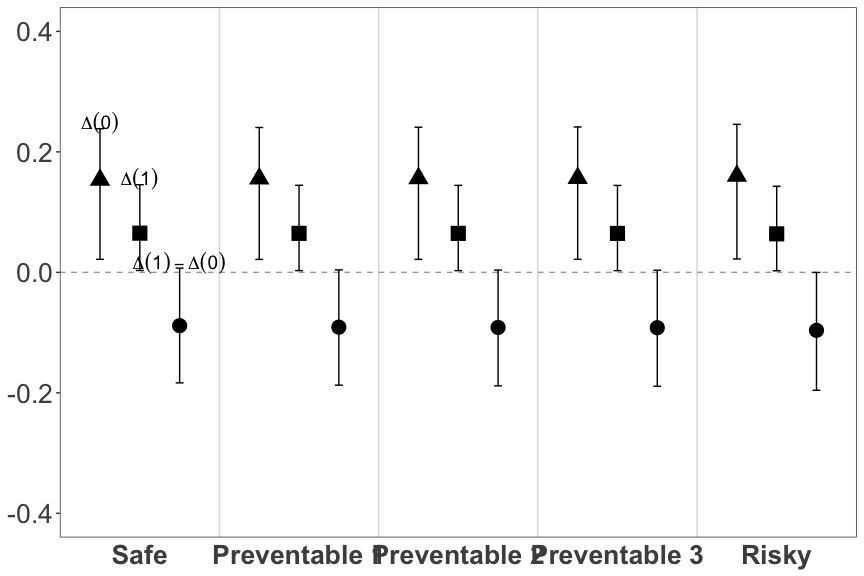### Replication codes To plot gender and racial fairness in Figure 5: # Fairness resfta1 = CalFairness(FTAace, attr = c(2,3)) resfta2 = CalFairness(FTAace, attr = c(4,5)) resnca1 = CalFairness(NCAace, attr = c(2,3)) resnca2 = CalFairness(NCAace, attr = c(4,5)) resnvca1 = CalFairness(NVCAace, attr = c(2,3)) resnvca2 = CalFairness(NVCAace, attr = c(4,5)) # Figure S5 p1 <- PlotFairness(resfta1, top.margin = 0.02, y.max = 0.3) + labs(title = "Failure to Appear (FTA)") + ylab("maximal sungroup difference") p4 <- PlotFairness(resfta2, top.margin = 0.02, y.max = 0.3) + labs(title = "Failure to Appear (FTA)") + ylab("maximal sungroup difference") p2 <- PlotFairness(resnca1, top.margin = 0.02, y.max = 0.3, label = F) + labs(title = "New Criminal Activity (NCA)") p5 <- PlotFairness(resnca2, top.margin = 0.02, y.max = 0.3, label = F) + labs(title = "New Criminal Activity (NCA)") p3 <- PlotFairness(resnvca1, top.margin = 0.02, y.max = 0.3, label = F) + labs(title = "New Violent Criminal Activity (NVCA)") p6 <- PlotFairness(resnvca2, top.margin = 0.02, y.max = 0.3, label = F) + labs(title = "New Violent Criminal Activity (NVCA)") pdf("figs/fair_gender.pdf", height = 5, width = 24) cowplot::plot_grid(p1,p2,p3, nrow = 1) dev.off() pdf("figs/fair_race.pdf", height = 5, width = 24) cowplot::plot_grid(p4,p5,p6, nrow = 1) dev.off() ## Optimal decision (Figure 6) CalOptimalDecision() conducts the following: 1. Calculate optimal decision for each observation given each of $$c_0$$ (cost of an outcome) and $$c_1$$ (cost of an unnecessarily harsh decision) from the lists. 2. Calculate difference in the expected utility between binary version of judge’s decisions and DMF recommendations given each of $$c_0$$ and $$c_1$$ from the lists. Note that the second component is used for the comparison between the judge’s decisions and DMF recommendations explained in the following section. The main inputs are as follows: • data: The same data.frame that is used for AiEvalmcmc(). • mcmc.re: The output of AiEvalmcmc(). • c0.ls: The list of possible cost of an outcome. • c1.ls: The list of possible cost of an unnecessarily harsh decision. You may also specify size, the number of parallel computing, based on the number of CPU cores. Also, if include.utility.diff.mcmc = TRUE, it would include Utility.diff.control.mcmc and Utility.diff.treated.mcmc in the output which is needed for the inference as in Figure S17. Please check the details with ?CalOptimalDecision before the analysis. Using the outputs of this function, we can visualize the results (estimated proportion of cases for which specific decision is optimal) by PlotOptimalDecision() as in Figure 6. The input is as follows: • If include.utility.diff.mcmc = FALSE, use the entire output of CalOptimalDecision(). • If include.utility.diff.mcmc = TRUE, use output$res.i.

Please check Section 3.7 and 4.4 for more details about the inference.

# ?CalOptimalDecision # Please check the details
synth_dmf = sample(0:1, nrow(synth), replace = TRUE) # random dmf recommendation
sample_optd_ci = CalOptimalDecision(data = synth,
mcmc.re = sample_mcmc,
c0.ls = seq(0,5,1), c1.ls = seq(0,5,1),
dmf = synth_dmf,
include.utility.diff.mcmc = TRUE,
# because of the above argument, the output is now a list
size = 2)
sample_optd = sample_optd_ci$res.i # Suppose we want to plot the proportion of cases for which *cash bond* # (either d1, d2 or d3) is optimal sample_optd$cash = sample_optd$d1 + sample_optd$d2 + sample_optd$d3 # Specify the column name of decision to be plotted, which is "cash" in this case. PlotOptimalDecision(sample_optd, "cash")  ### Replication codes To plot estimated proportion of cases for which cash bond is optimal as in Figure 6 (and Figure S16): # Optimal Decision optd_fta_ci = CalOptimalDecision(data = FTAdata, mcmc.re = FTAmcmc, c0.ls = seq(0,10,0.5), c1.ls = seq(0,3,0.1), dmf = PSAdata$DMF,  size = 5,
include.utility.diff.mcmc = TRUE)
optd_nca_ci = CalOptimalDecision(data = NCAdata, mcmc.re = NCAmcmc,
c0.ls = seq(0,10,0.5), c1.ls = seq(0,3,0.1),
dmf = PSAdata$DMF, size = 5, include.utility.diff.mcmc = TRUE) optd_nvca_ci = CalOptimalDecision(data = NVCAdata, mcmc.re = NVCAmcmc, c0.ls = seq(0,10,0.5), c1.ls = seq(0,3,0.1), dmf = PSAdata$DMF,  size = 5,
include.utility.diff.mcmc = TRUE)
optd_fta = optd_fta_ci$res.i optd_nca = optd_nca_ci$res.i
optd_nvca = optd_nvca_ci$res.i optd_fta$cash = optd_fta$d1 + optd_fta$d2
optd_nca$cash = optd_nca$d1 + optd_nca$d2 optd_nvca$cash = optd_nvca$d1 + optd_nvca$d2

# Figure 6
p1 <- PlotOptimalDecision(res = optd_fta, colname.d = "cash", idx = which(PSAdata$DMF == 0)) + labs(title = "Failure to Appear (FTA)", x = expression("Cost of FTA ("*c*")"), y = expression("Cost of unnecessarily harsh decision ("*c*")")) p2 <- PlotOptimalDecision(res = optd_nca, colname.d = "cash", idx = which(PSAdata$DMF == 0)) +
labs(title = "New Criminal Activity (NCA)", x = expression("Cost of NCA ("*c*")"), y = NULL)
p3 <- PlotOptimalDecision(res = optd_nvca, colname.d = "cash", idx = which(PSAdata$DMF == 0), label.place = label_placement_fraction(0.9)) + labs(title = "New Violent Criminal Activity (NVCA)", x = expression("Cost of NVCA ("*c*")"), y = NULL) p4 <- PlotOptimalDecision(res = optd_fta, colname.d = "cash", idx = which(PSAdata$DMF == 1)) +
labs(x = expression("Cost of FTA ("*c*")"),
y = expression("Cost of unnecessarily harsh decision ("*c*")"))
p5 <- PlotOptimalDecision(res = optd_nca, colname.d = "cash", idx = which(PSAdata$DMF == 1)) + labs(x = expression("Cost of NCA ("*c*")"), y =NULL) p6 <- PlotOptimalDecision(res = optd_nvca, colname.d = "cash", idx = which(PSAdata$DMF == 1),
label.place = label_placement_fraction(0.9)) +
labs(x = expression("Cost of NVCA ("*c*")"), y = NULL)

pdf("figs/optd_combined_signature.pdf", width = 16, height = 5.2)
cowplot::plot_grid(p1, p2, p3, nrow = 1)
dev.off()

pdf("figs/optd_combined_cash.pdf", width = 16, height = 5.2)
cowplot::plot_grid(p4, p5, p6, nrow = 1)
dev.off()

# Figure S16
p1 <- PlotOptimalDecision(res = optd_fta, colname.d = "cash", idx = which(PSAdata$FTAScore<=4)) + labs(title = "Failure to Appear (FTA)", subtitle = "FTA Score: 1 - 4", x = expression("Cost of FTA ("*c*")"), y = expression("Cost of unnecessarily harsh decision ("*c*")")) p2 <- PlotOptimalDecision(res = optd_nca, colname.d = "cash", idx = which(PSAdata$NCAScore<=4)) +
labs(title = "New Criminal Activity (NCA)", subtitle = "NCA Score: 1 - 4",
x = "NCA Score: 1 - 4", y = NULL)
p3 <- PlotOptimalDecision(res = optd_nvca, colname.d = "cash", idx = which(PSAdata$NVCAFlag==0), label.place = label_placement_fraction(0.9)) + labs(title = "New Violent Criminal Activity (NVCA)", subtitle = "NVCA Flag: 0", x = expression("Cost of NVCA ("*c*")"), y = NULL) p4 <- PlotOptimalDecision(res = optd_fta, colname.d = "cash", idx = which(PSAdata$FTAScore>4)) +
labs(subtitle = "FTA Score: 5 - 6",  x = expression("Cost of FTA ("*c*")"),
y = expression("Cost of unnecessarily harsh decision ("*c*")"))
p5 <- PlotOptimalDecision(res = optd_nca, colname.d = "cash", idx = which(PSAdata$NCAScore>4)) + labs(subtitle = "NCA Score: 5 - 6", x = expression("Cost of NCA ("*c*")"), y =NULL) p6 <- PlotOptimalDecision(res = optd_nvca, colname.d = "cash", idx = which(PSAdata$NVCAFlag==1),
label.place = label_placement_fraction(0.9)) +
labs(subtitle = "NVCA Flag: 1", x = expression("Cost of NVCA ("*c*")"), y = NULL)

pdf("figs/optd_separate_signature.pdf", width = 16, height = 5.2)
cowplot::plot_grid(p1, p2, p3, nrow = 1)
dev.off()

pdf("figs/optd_separate_cash.pdf", width = 16, height = 5.2)
cowplot::plot_grid(p4, p5, p6, nrow = 1)
dev.off()

## Comparison between the judge’s decisions and DMF recommendations (Figure 7)

Using the output of CalOptimalDecision(), we can compare the judge’s decisions and DMF recommendations. See Section 4.5 for more details. PlotUtilityDiff() visualizes the estimated difference in the expected utility between judge’s decisions and DMF Recommendations (e.g., Figure 7). Additionally, PlotUtilityDiffCI() plot the estimated difference with 95% confidence interval (e.g., Figure S17).

PlotUtilityDiff(sample_optd_ci$res.i) PlotUtilityDiffCI(sample_optd_ci$res.mcmc)

### Replication codes

Codes for Figure 7 and Figure S17:

# Figure 7
p1 <- PlotUtilityDiff(optd_fta, which(PSAdata$Z==1)) + labs(title = "Failure to Appear (FTA)", x = expression("Cost of FTA ("*c*")"), y = expression("Cost of unnecessarily harsh decision ("*c*")")) p2 <- PlotUtilityDiff(optd_nca, which(PSAdata$Z==1)) +
labs(title = "New Criminal Activity (NCA)",
x = expression("Cost of NCA ("*c*")"), y = NULL)
p3 <- PlotUtilityDiff(optd_nvca, which(PSAdata$Z==1)) + labs(title = "New Violent Criminal Activity (NVCA)", x = expression("Cost of NVCA ("*c*")"), y = NULL) p4 <- PlotUtilityDiff(optd_fta, which(PSAdata$Z==0)) +
labs(x = expression("Cost of FTA ("*c*")"),
y = expression("Cost of unnecessarily harsh decision ("*c*")"))
p5 <- PlotUtilityDiff(optd_nca, which(PSAdata$Z==0)) + labs(x = expression("Cost of NCA ("*c*")"), y =NULL) p6 <- PlotUtilityDiff(optd_nvca, which(PSAdata$Z==0)) +
labs(x = expression("Cost of NVCA ("*c*")"), y = NULL)

pdf("figs/utility_treatment.pdf", width = 16, height = 5.2)
cowplot::plot_grid(p1, p2, p3, nrow = 1)
dev.off()

pdf("figs/utility_control.pdf", width = 16, height = 5.2)
cowplot::plot_grid(p4, p5, p6, nrow = 1)
dev.off()

# Figure S17
p.fta <- PlotUtilityDiffCI(optd_fta_ci$res.mcmc) p.nca <- PlotUtilityDiffCI(optd_nca_ci$res.mcmc)
p.nvca <- PlotUtilityDiffCI(optd_nvca_ci$res.mcmc) p1 <- p.fta$p.treated + labs(title = "Failure to Appear (FTA)",
y = "Difference in the Expected Utility",
x = expression("Cost of FTA ("*c*")"))
p2 <- p.nca$p.treated + labs(title = "New Criminal Activity (NCA)", y = "Difference in the Expected Utility", x = expression("Cost of NCA ("*c*")")) p3 <- p.nvca$p.treated + labs(title = "New Violent Criminal Activity (NVCA)",
y = "Difference in the Expected Utility",
x = expression("Cost of NVCA ("*c*")"))
p4 <- p.fta$p.control + labs(title = "Failure to Appear (FTA)", y = "Difference in the Expected Utility", x = expression("Cost of FTA ("*c*")")) p5 <- p.nca$p.control + labs(title = "New Criminal Activity (NCA)",
y = "Difference in the Expected Utility",
x = expression("Cost of NCA ("*c*")"))
p6 <- p.nvca$p.control + labs(title = "New Violent Criminal Activity (NVCA)", y = "Difference in the Expected Utility", x = expression("Cost of NVCA ("*c*")")) pdf("figs/utility_treat_ci.pdf", width = 18, height = 6) cowplot::plot_grid(p1, p2, p3, nrow = 1) dev.off() pdf("figs/utility_control_ci.pdf", width = 18, height = 6) cowplot::plot_grid(p4, p5, p6, nrow = 1) dev.off() ## Test of spillover effects: Conditional Randomization Test (CRT) In Appendix S3, we examine the possible existence of spillover effects using CRT. SpilloverCRT() conducts CRT based on court hearing date and additionally SpilloverCRTpower() conduct power analysis of the test using simulation. The result can be visualized using PlotSpilloverCRT() and PlotSpilloverCRTpower(). See the example below. # CRT data(hearingdate_synth) crt <- SpilloverCRT(D = synth$D,
Z = synth$Z, CourtEvent_HearingDate = hearingdate_synth, n = 100) # increase the permutation size to 10000 PlotSpilloverCRT(crt) # Power analysis crt_power <- SpilloverCRTpower(D = synth$D,
Z = synth$Z, CourtEvent_HearingDate = hearingdate_synth) PlotSpilloverCRTpower(crt_power) ### Replication codes Codes for Figure S8 and Figure S9: data(HearingDate) crt_data <- data.frame( D = FTAdata$D,
Z = FTAdata$Z, CourtEvent_HearingDate = HearingDate ) # CRT crt <- SpilloverCRT(D = crt_data$D,
Z = crt_data$Z, CourtEvent_HearingDate = crt_data$CourtEvent_HearingDate,
n = 10000)
# Figure S8
pdf("figs/hist_crt.pdf", width = 4, height = 6)
PlotSpilloverCRT(crt)
dev.off()

# Power analysis
crt_power <- SpilloverCRTpower(D = crt_data$D, Z = crt_data$Z,
CourtEvent_HearingDate = crt_data$CourtEvent_HearingDate, size = 5, n = 1000, m = 1000, cand_omegaZtilde = seq(-1.5, 1.5, by = 0.5)) # Figure S9 pdf("figs/crt_power_prop.pdf", width = 4, height = 6) PlotSpilloverCRTpower(crt_power) dev.off() ## Frequentist analysis In Appendix S7, we implement frequentist analysis and present the results. This can be done by CalAPCEipw(). Additionally, you may have to run BootstrapAPCEipw() for the confidence interval. Note that you should run CalAPCEipw() for each of subgroups, and make sure to drop the column that is constant across a specific subgroup (e.g., Sex column for the subset of Female arrestees). The result can be summarized with APCEsummaryipw() and visualized by PlotAPCE() as before. synth$SexWhite = synth$Sex * synth$White
freq_apce = CalAPCEipw(synth)
boot_apce = BootstrapAPCEipw(synth, rep = 10)
# subgroup analysis
data_s0 = subset(synth, synth$Sex==0,select=-c(Sex,SexWhite)) freq_s0 = CalAPCEipw(data_s0) boot_s0 = BootstrapAPCEipw(data_s0, rep = 10) data_s1 = subset(synth, synth$Sex==1,select=-c(Sex,SexWhite))
freq_s1 = CalAPCEipw(data_s1)
boot_s1 = BootstrapAPCEipw(data_s1, rep = 10)
data_s1w0 = subset(synth, synth$Sex==1&synth$White==0,select=-c(Sex,White,SexWhite))
freq_s1w0 = CalAPCEipw(data_s1w0)
boot_s1w0 = BootstrapAPCEipw(data_s1w0, rep = 10)
data_s1w1 = subset(synth, synth$Sex==1&synth$White==1,select=-c(Sex,White,SexWhite))
freq_s1w1 = CalAPCEipw(data_s1w1)
boot_s1w1 = BootstrapAPCEipw(data_s1w1, rep = 10)

freq_apce_summary <- APCEsummaryipw(freq_apce, freq_s0, freq_s1,
freq_s1w0, freq_s1w1,
boot_apce, boot_s0, boot_s1,
boot_s1w0, boot_s1w0)
PlotAPCE(freq_apce_summary, y.max = 0.25,
decision.labels = c("signature","small cash","middle cash","large cash"),
shape.values = c(16, 17, 15, 18),
col.values = c("blue", "black", "red", "brown", "purple"), label = FALSE)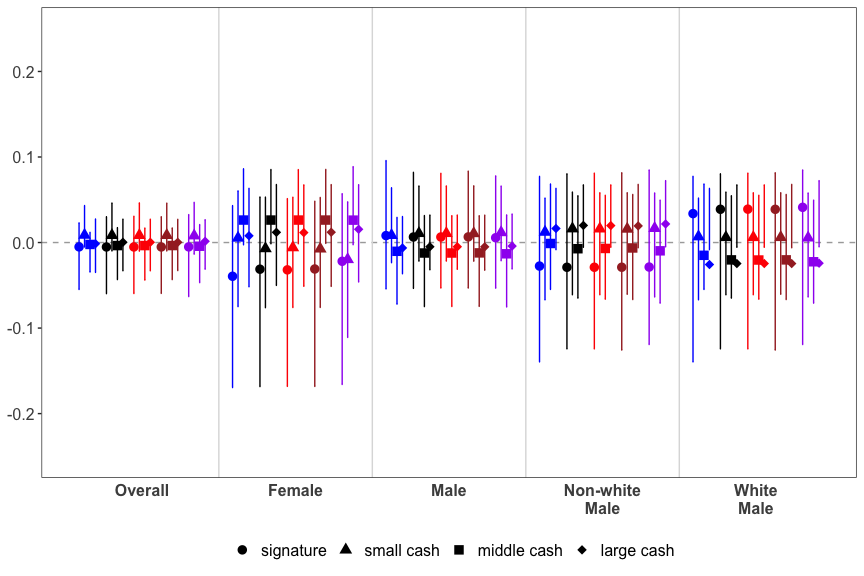We also fit the model including random effects for the hearing date of the case in the paper. This can be done with CalAPCEipwRE() and BootstrapAPCEipwRE()/BootstrapAPCEipwREparallel(). You may need to specify the precise formula of the model in this case.

### Replication codes

Codes for Figure S10, S11, and S12.

set.seed(123) # seed for bootstrap
## Frequentist approach
# FTA
data_s0 = subset(FTAdata, FTAdata$Sex==0,select=-c(Sex,SexWhite)) freq_s0 = CalAPCEipw(data_s0) boot_s0 = BootstrapAPCEipw(data_s0, rep = 1000) data_s1 = subset(FTAdata, FTAdata$Sex==1,select=-c(Sex,SexWhite))
freq_s1 = CalAPCEipw(data_s1)
boot_s1 = BootstrapAPCEipw(data_s1, rep = 1000)
data_s1w0 = subset(FTAdata, FTAdata$Sex==1&FTAdata$White==0,select=-c(Sex,White,SexWhite))
freq_s1w0 = CalAPCEipw(data_s1w0)
boot_s1w0 = BootstrapAPCEipw(data_s1w0, rep = 1000)
data_s1w1 = subset(FTAdata, FTAdata$Sex==1&FTAdata$White==1,select=-c(Sex,White,SexWhite))
freq_s1w1 = CalAPCEipw(data_s1w1)
boot_s1w1 = BootstrapAPCEipw(data_s1w1, rep = 1000)
fta_apce_summary <- APCEsummaryipw(freq_apce, freq_s0, freq_s1,
freq_s1w0, freq_s1w1,
boot_apce, boot_s0, boot_s1,
boot_s1w0, boot_s1w0)
# NCA
data_s0 = subset(NCAdata, NCAdata$Sex==0,select=-c(Sex,SexWhite)) freq_s0 = CalAPCEipw(data_s0) boot_s0 = BootstrapAPCEipw(data_s0, rep = 1000) data_s1 = subset(NCAdata, NCAdata$Sex==1,select=-c(Sex,SexWhite))
freq_s1 = CalAPCEipw(data_s1)
boot_s1 = BootstrapAPCEipw(data_s1, rep = 1000)
data_s1w0 = subset(NCAdata, NCAdata$Sex==1&NCAdata$White==0,select=-c(Sex,White,SexWhite))
freq_s1w0 = CalAPCEipw(data_s1w0)
boot_s1w0 = BootstrapAPCEipw(data_s1w0, rep = 1000)
data_s1w1 = subset(NCAdata, NCAdata$Sex==1&NCAdata$White==1,select=-c(Sex,White,SexWhite))
freq_s1w1 = CalAPCEipw(data_s1w1)
boot_s1w1 = BootstrapAPCEipw(data_s1w1, rep = 1000)
nca_apce_summary <- APCEsummaryipw(freq_apce, freq_s0, freq_s1,
freq_s1w0, freq_s1w1,
boot_apce, boot_s0, boot_s1,
boot_s1w0, boot_s1w0)

# Figure S10
pdf("figs/qoi_fta_freq.pdf",width = 9, height = 3)
PlotAPCE(fta_freq_apce_summary, y.max = 0.23, top.margin = 0.05, bottom.margin = 0.05,
label.position = c("top", "bottom", "top", "bottom")) +
labs(title = "Failure to Appear (FTA)") +
theme(legend.position = "none")
dev.off()
pdf("figs/qoi_nca_freq.pdf",width = 9, height = 3.5)
PlotAPCE(nca_freq_apce_summary, y.max = 0.23, label = FALSE) +
labs(title = "New Criminal Activity (NCA)")
dev.off()

## Random effects
full.demographics = "Sex + White + SexWhite + Age"
full.other.cov = "PendingChargeAtTimeOfOffense + NCorNonViolentMisdemeanorCharge + ViolentMisdemeanorCharge + ViolentFelonyCharge + NonViolentFelonyCharge + PriorMisdemeanorConviction + PriorFelonyConviction + PriorViolentConviction + PriorSentenceToIncarceration + PriorFTAInPastTwoYears + PriorFTAOlderThanTwoYears + Staff_ReleaseRecommendation + (1|CourtEvent_HearingDate) + D"

mod = paste0("Y ~ ", full.demographics, " + ", full.other.cov)
mod.s = paste0("Y ~ White + Age + ", full.other.cov)
mod.sw = paste0("Y ~ Age + ", full.other.cov)

# FTA
freq_apce = CalAPCEipwRE(FTAdata, formula = mod)
boot_apce = BootstrapAPCEipwRE(FTAdata, rep=1000, formula = mod)
data_s0 = subset(FTAdata, FTAdata$Sex==0,select=-c(Sex,SexWhite)) freq_s0 = CalAPCEipwRE(data_s0, formula = mod.s) boot_s0 = BootstrapAPCEipwRE(data_s0, rep = 1000, formula = mod.s) data_s1 = subset(FTAdata, FTAdata$Sex==1,select=-c(Sex,SexWhite))
freq_s1 = CalAPCEipwRE(data_s1, formula = mod.s)
boot_s1 = BootstrapAPCEipwRE(data_s1, rep = 1000, formula = mod.s)
data_s1w0 = subset(FTAdata, FTAdata$Sex==1&FTAdata$White==0,select=-c(Sex,White,SexWhite))
freq_s1w0 = CalAPCEipwRE(data_s1w0, formula = mod.sw)
boot_s1w0 = BootstrapAPCEipwRE(data_s1w0, rep = 1000, formula = mod.sw)
data_s1w1 = subset(FTAdata, FTAdata$Sex==1&FTAdata$White==1,select=-c(Sex,White,SexWhite))
freq_s1w1 = CalAPCEipwRE(data_s1w1, formula = mod.sw)
boot_s1w1 = BootstrapAPCEipwRE(data_s1w1, rep = 1000, formula = mod.sw)
fta_re_apce_summary <- APCEsummaryipw(freq_apce, freq_s0, freq_s1,
freq_s1w0, freq_s1w1,
boot_apce, boot_s0, boot_s1,
boot_s1w0, boot_s1w0)
# NCA
freq_apce = CalAPCEipwRE(NCAdata, formula = mod)
boot_apce = BootstrapAPCEipwRE(NCAdata, rep=1000, formula = mod)
data_s0 = subset(NCAdata, NCAdata$Sex==0,select=-c(Sex,SexWhite)) freq_s0 = CalAPCEipwRE(data_s0, formula = mod.s) boot_s0 = BootstrapAPCEipwRE(data_s0, rep = 1000, formula = mod.s) data_s1 = subset(NCAdata, NCAdata$Sex==1,select=-c(Sex,SexWhite))
freq_s1 = CalAPCEipwRE(data_s1, formula = mod.s)
boot_s1 = BootstrapAPCEipwRE(data_s1, rep = 1000, formula = mod.s)
data_s1w0 = subset(NCAdata, NCAdata$Sex==1&NCAdata$White==0,select=-c(Sex,White,SexWhite))
freq_s1w0 = CalAPCEipwRE(data_s1w0, formula = mod.sw)
boot_s1w0 = BootstrapAPCEipwRE(data_s1w0, rep = 1000, formula = mod.sw)
data_s1w1 = subset(NCAdata, NCAdata$Sex==1&NCAdata$White==1,select=-c(Sex,White,SexWhite))
freq_s1w1 = CalAPCEipwRE(data_s1w1, formula = mod.sw)
boot_s1w1 = BootstrapAPCEipwRE(data_s1w1, rep = 1000, formula = mod.sw)
nca_re_apce_summary <- APCEsummaryipw(freq_apce, freq_s0, freq_s1,
freq_s1w0, freq_s1w1,
boot_apce, boot_s0, boot_s1,
boot_s1w0, boot_s1w0)

# Figure S11
pdf("figs/qoi_fta_freq_re.pdf",width = 9, height = 3)
PlotAPCE(fta_freq_apce_summary, y.max = 0.34, top.margin = 0.1, bottom.margin = 0.1
label.position = c("top", "bottom", "top", "bottom")) +
labs(title = "Failure to Appear (FTA)") +
theme(legend.position = "none")
dev.off()
pdf("figs/qoi_nca_freq_re.pdf",width = 9, height = 3.5)
PlotAPCE(nca_freq_apce_summary, y.max = 0.34, label = FALSE) +
labs(title = "New Criminal Activity (NCA)")
dev.off()

## Age subgroup
# FTA
data_age1 = subset(FTAdata, FTAdata$Age<=22) freq_age1 = CalAPCEipw(data_age1) boot_age1 = BootstrapAPCEipw(data_age1, rep = 1000) data_age2 = subset(FTAdata, FTAdata$Age>22 & FTAdata$Age<=28) freq_age2 = CalAPCEipw(data_age2) boot_age2 = BootstrapAPCEipw(data_age2, rep = 1000) data_age3 = subset(FTAdata, FTAdata$Age>28 & FTAdata$Age<=35) freq_age3 = CalAPCEipw(data_age3) boot_age3 = BootstrapAPCEipw(data_age3, rep = 1000) data_age4 = subset(FTAdata, FTAdata$Age>35 & FTAdata$Age<=45) freq_age4 = CalAPCEipw(data_age4) boot_age4 = BootstrapAPCEipw(data_age4, rep = 1000) data_age5 = subset(FTAdata, FTAdata$Age>45)
freq_age5 = CalAPCEipw(data_age5)
boot_age5 = BootstrapAPCEipw(data_age5, rep = 1000)
fta_apce_summary <- APCEsummaryipw(freq_age1, freq_age2,freq_age3,
freq_age4, freq_age5,
boot_age1, boot_age2,boot_age3,
boot_age4, boot_age5)
# NCA
data_age1 = subset(NCAdata, NCAdata$Age<=22) freq_age1 = CalAPCEipw(data_age1) boot_age1 = BootstrapAPCEipw(data_age1, rep = 1000) data_age2 = subset(NCAdata, NCAdata$Age>22 & NCAdata$Age<=28) freq_age2 = CalAPCEipw(data_age2) boot_age2 = BootstrapAPCEipw(data_age2, rep = 1000) data_age3 = subset(NCAdata, NCAdata$Age>28 & NCAdata$Age<=35) freq_age3 = CalAPCEipw(data_age3) boot_age3 = BootstrapAPCEipw(data_age3, rep = 1000) data_age4 = subset(NCAdata, NCAdata$Age>35 & NCAdata$Age<=45) freq_age4 = CalAPCEipw(data_age4) boot_age4 = BootstrapAPCEipw(data_age4, rep = 1000) data_age5 = subset(NCAdata, NCAdata$Age>45)
freq_age5 = CalAPCEipw(data_age5)
boot_age5 = BootstrapAPCEipw(data_age5, rep = 1000)
fta_apce_summary <- APCEsummaryipw(freq_age1, freq_age2,freq_age3,
freq_age4, freq_age5,
boot_age1, boot_age2,boot_age3,
boot_age4, boot_age5)
# Figure S12
pdf("figs/qoi_fta_freq_age.pdf",width = 9, height = 3)
PlotAPCE(fta_freq_apce_summary, y.max = 0.25, top.margin = 0.13, bottom.margin = 0.09,
label.position = c("top", "bottom", "top", "bottom"),
name.group = c("17~22", "23~28", "29~35", "36~45", "46~")) +
labs(title = "Failure to Appear (FTA)") +
theme(legend.position = "none")
dev.off()
pdf("figs/qoi_nca_freq_age.pdf",width = 9, height = 3.5)
PlotAPCE(nca_freq_apce_summary, y.max = 0.25, label = FALSE,
name.group = c("17~22", "23~28", "29~35", "36~45", "46~")) +
labs(title = "New Criminal Activity (NCA)")
dev.off()

## Sensitivity analysis

As discussed in Section 3.5, we conduct sensitivity analysis of the unconfoundedness assumption, which may be violated when researchers do not observe some information that is used by the judge and is predictive of arrestees’ behavior. Parametric sensitivity analysis can be done by setting non-zero rho, the sensitivity parameter, for AiEvalmcmc() and CalAPCE()/CalAPCEparallel().

### Replication codes

Codes for Figure S13, S14, and S15.

## rho = 0.05
## Main analysis
# MCMC

# APCE
FTAqoi <- APCEsummary(FTAace[["APCE.mcmc"]])

NCAqoi <- APCEsummary(NCAace[["APCE.mcmc"]])

NVCAqoi <- APCEsummary(NVCAace[["APCE.mcmc"]])

# Figure S13
pdf("figs/sens_fta_005.pdf", width = 9, height = 3)
PlotAPCE(FTAqoi, y.max = 0.18, top.margin = 0.05, bottom.margin = 0.05,
label.position = c("top", "bottom", "top", "bottom")) +
labs(title = "Failure to Appear (FTA)", subtitle = expression(paste(rho, "= 0.05"))) +
theme(legend.position = "none")
dev.off()
pdf("figs/sens_nca_005.pdf", width = 9, height = 3)
PlotAPCE(NCAqoi, y.max = 0.18, label = FALSE) +
labs(title = "New Criminal Activity (NCA)", subtitle = expression(paste(rho, "= 0.05"))) +
theme(legend.position = "none")
dev.off()
pdf("figs/sens_nvca_005.pdf", width = 9, height = 3.5)
PlotAPCE(NVCAqoi, y.max = 0.3, label = FALSE) +
labs(title = "New Violent Criminal Activity (NVCA)", subtitle = expression(paste(rho, "= 0.05")))
dev.off()

## rho = 0.1
## Main analysis
# MCMC

# APCE
FTAqoi <- APCEsummary(FTAace[["APCE.mcmc"]])

NCAqoi <- APCEsummary(NCAace[["APCE.mcmc"]])

NVCAqoi <- APCEsummary(NVCAace[["APCE.mcmc"]])

# Figure S14
pdf("figs/sens_fta_01.pdf", width = 9, height = 3)
PlotAPCE(FTAqoi, y.max = 0.19, top.margin = 0.05, bottom.margin = 0.05,
label.position = c("top", "bottom", "top", "bottom")) +
labs(title = "Failure to Appear (FTA)", subtitle = expression(paste(rho, "= 0.1"))) +
theme(legend.position = "none")
dev.off()
pdf("figs/sens_nca_01.pdf", width = 9, height = 3)
PlotAPCE(NCAqoi, y.max = 0.19, label = FALSE) +
labs(title = "New Criminal Activity (NCA)", subtitle = expression(paste(rho, "= 0.1"))) +
theme(legend.position = "none")
dev.off()
pdf("figs/sens_nvca_01.pdf", width = 9, height = 3.5)
PlotAPCE(NVCAqoi, y.max = 0.38, label = FALSE) +
labs(title = "New Violent Criminal Activity (NVCA)", subtitle = expression(paste(rho, "= 0.1")))
dev.off()

## rho = 0.3
## Main analysis
# MCMC

# APCE
FTAqoi <- APCEsummary(FTAace[["APCE.mcmc"]])

NCAqoi <- APCEsummary(NCAace[["APCE.mcmc"]])

NVCAqoi <- APCEsummary(NVCAace[["APCE.mcmc"]])

# Figure S15
pdf("figs/sens_fta_03.pdf", width = 9, height = 3)
PlotAPCE(FTAqoi, y.max = 0.16, top.margin = 0.05, bottom.margin = 0.05,
label.position = c("top", "bottom", "top", "bottom")) +
labs(title = "Failure to Appear (FTA)", subtitle = expression(paste(rho, "= 0.3"))) +
theme(legend.position = "none")
dev.off()
pdf("figs/sens_nca_03.pdf", width = 9, height = 3)
PlotAPCE(NCAqoi, y.max = 0.16, label = FALSE) +
labs(title = "New Criminal Activity (NCA)", subtitle = expression(paste(rho, "= 0.3"))) +
theme(legend.position = "none")
dev.off()
pdf("figs/sens_nvca_03.pdf", width = 9, height = 3.5)
PlotAPCE(NVCAqoi, y.max = 0.62, label = FALSE) +
labs(title = "New Violent Criminal Activity (NVCA)", subtitle = expression(paste(rho, "= 0.3")))
dev.off()